# GRE Math Multi-subject Challenge Question

Once in a while it is fun to take a concept in an official GRE question and run with it. The question below is inspired by a question from the 2nd edition Official Guide. That is not to say it is that similar; I have only extracted a couple of concepts from that problem.

I’ve also made my question far more difficult. The first question was already difficult enough, so it is safe to say that a question of this difficulty would never appear on the GRE (though it could appear on the GMAT). Another of way expressing this: only attempt this question if you are already very strong in Quant. If not, just have fun with it, remembering that questions on the actual GRE will be easier.

1. A semicircle with area of xπ is marked by seven points equally spaced along the half arc of the semicircle, such that two of the seven points form the endpoints of the diameter. What is the probability of forming a triangle with an area less than x from the total number of triangles formed by combining two of the seven points and the center of the diameter?

1. 4/5
2. 6/7
3. 17/21
4. 19/21
5. 31/35

Again this is a very difficult question, one that requires many steps, and one that has numerous twists. The question is without a doubt expansive, covering in its sweep combinatorics, probability, basic geometry, advanced triangle theory, counting properties and much more. So hang on for the ride!

## Step #1 – Find area of circle in terms of x

In order to deal with this whole area of the triangle business, we first have to figure out the area of the circle. That way, we can also derive the radius, a number that be instrumental in helping us in Step #3.

First though we find the area of the circle, which is twice that of the semi-circle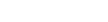. To solve for the radius, we use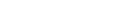.

## Step #2 – Figure out the whole seven points issue

So we have the radius. But how does that help in figuring out the resulting area of any of the inscribed triangles? Well, remember the seven points? If two points are different sides of the diameter and the other five points are evenly spaced along the arc, then the semicircle is broken into equal sixths. Therefore the central angle of each will be 30 degrees (the arc of a semicircle corresponds to 180 degrees).

## Step #3 – Find the range of the areas of possible inscribed triangles

Using the information above, we can start to play around with triangles, keeping in mind that any two points along the arc will form radii with the center of the diameter (remember that the original question asked us to make triangles using the middle of the diameter).

A good idea is to come up with a triangle that both meets the criteria and is easy to solve area-wise. That way you can see how close you are to an area of x. For instance, you could assume that the triangle is an equilateral, the two points on the arc a distance of 60 degrees away (and thus the central angle will be 60).

A radius of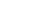will form the sides of the equilateral. Using the formula for the area of the equilateral triangle,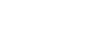, where s equals the side of the triangle, or in this case the radius, we get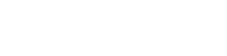. Because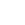equals approximately 1.7, we can see that such an equilateral triangle has an area less than x.

But don’t give up just yet. In trying to increase the area you should keep in mind that skinnier triangles, say with points on the opposite side of the semicircle, will not have an area greater than that of an equilateral. So we want to increase the angle but not too much.

The next most sensible triangle—that is one in which we can use simple formulas to determine the area—is a 45:45:90 triangle, which means that the two points on the semicircle will have to be 90 degrees apart (that way the central angle will be 90 degrees. And with two equal sides (remember all radii are equal), we know have a 45:45:90 triangle with sides of. Using the formula for the area of a triangle, we get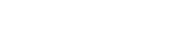.

An important insight: if we bring the points together, we end up with an equilateral, which we know to have a smaller area. Therefore, moving the points on the arc together results in a triangle with less area. Moving the points apart makes the triangle skinnier, something that also results in a triangle with less area.

Therefore, the isosceles right triangle represents the largest possible triangle, area-wise, that you can inscribe given the conditions of the problem (pretty advanced stuff, right?).

## Step #4 – Find the total number of triangles

Voila! Finally, we have it. The only possible triangle to yield x will be an isosceles right triangle, or a 45-45-90, with a central angle of 90. If we find the number of these triangles and subtract that from 1, we have the probability of choosing a triangle with an area less than 1.

The total number of isosceles right triangle is four, which we can find by choosing points along the circle that are 90 arc degrees apart.

Next we want to find the total number of triangles. Because one point is already fixed (the center of the diameter), we have to determine how many triangles can be formed by using two of the seven points. The ordering of the vertices is not important. Meaning that a triangle with points A, B, and C, is the same as triangle with points B, C, A. Therefore we use 7C2 = 21.

## Step #5 – Don’t count the line!

Just when you thought we were finally done with this monstrosity of the problem, there is one final, and subtle twist. We have to discount one of these twenty-one triangles because it is actually not a triangle. If we choose both end points of the diameter and the center of the diameter, we have a straight line (this line is the diameter of the semicircle).

Therefore, there are twenty triangles, sixteen of which have an area less than x. so the answer is (A) 4/5.

## Author

•Chris Lele is the Principal Curriculum Manager (and vocabulary wizard) at Magoosh. Chris graduated from UCLA with a BA in Psychology and has 20 years of experience in the test prep industry. He's been quoted as a subject expert in many publications, including US News, GMAC, and Business Because. In his time at Magoosh, Chris has taught countless students how to tackle the GRE, GMAT, SAT, ACT, MCAT (CARS), and LSAT exams with confidence. Some of his students have even gone on to get near-perfect scores. You can find Chris on YouTube, LinkedIn, Twitter and Facebook!